# Use Stokes' Theorem to evaluate  F · dr where C is oriented counterclockwise as viewed from above.F(x, y, z) = (x + y2)i + (y + z2)j + (z + x2)k,C is the triangle with vertices (3, 0, 0), (0, 3, 0), and (0, 0, 3).

Question
36 views

Use Stokes' Theorem to evaluate

F · dr

where C is oriented counterclockwise as viewed from above.

F(x, y, z) = (x + y2)i + (y + z2)j + (z + x2)k,

C is the triangle with vertices
(3, 0, 0), (0, 3, 0), and (0, 0, 3).
check_circle

Step 1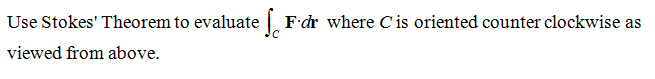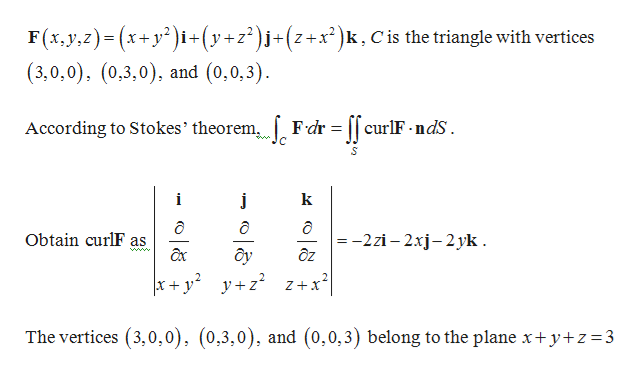help_outlineImage TranscriptioncloseF(x, y,z)= (x+y² )i+(y+z² )j+(z +x² )k , C is the triangle with vertices (3,0,0), (0,3,0), and (0,0,3). Fdr = [[ curlF - ndS. According to Stokes' theorem, i k = -2zi – 2xj-2 yk . дz Obtain curlF as ôy x+ y y+z z+x² The vertices (3,0,0), (0,3,0), and (0,0,3) belong to the plane x+ y+z=3 fullscreen
Step 2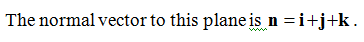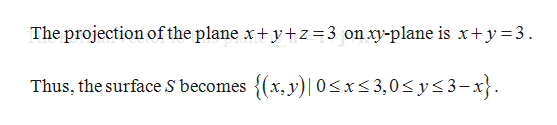help_outlineImage TranscriptioncloseThe projection of the plane x+y+z=3 onxy-plane is x+y=3. Thus, the surface S becomes {(x,y)|0
Step 3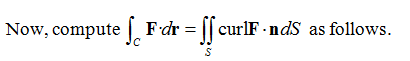...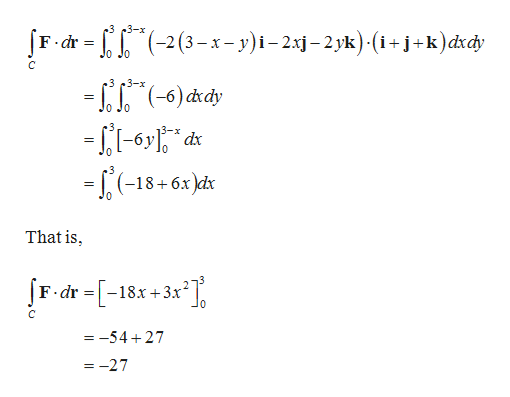help_outlineImage Transcriptionclose-33-x [F- dr = (-2(3 – x – y)i– 2xj– 2yk)·(i+j+k)dxdy Jo Jo .3 3-x - "(-6) xdy -LI-6y" dx - ,(-18+ 6x )kr 3-x %3D That is, F dr =[-18x+3x² = -54+27 = -27 fullscreen

### Want to see the full answer?

See Solution

#### Want to see this answer and more?

Solutions are written by subject experts who are available 24/7. Questions are typically answered within 1 hour.*

See Solution
*Response times may vary by subject and question.
Tagged in

### Calculus